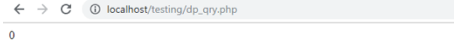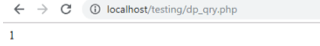Difference Between != and !== in PHP# Difference Between != and !== in PHP

4062

Hey You,

How are you going today !

You know, while I was teaching my students about PHP operators today, one of my students asked about the difference between != and !== operator and assumed that almost both of the operators worked similarly.

That rang a bell in my head and I decided why not just help my students but also everyone who is confused with the working of these two operators in PHP. So, here I am with my informative blog on the difference between != and !== in PHP.

Note that you can also Learn PHP Online to know more about these operators.

Operators in PHP

Without moving further, let us first understand what PHP operators are and what are they used for!

You must have performed various operations while writing code, be it arithmetic or logical operations. However, to instruct the program to perform a certain operation on the variables, we need operators. These operators can be termed as symbols that perform any operation on the variable. For instance, let us look at some of the operators which are used in PHP:

+: for adding two variables (a + b = x;)

-: for subtracting a variable from another (a - b = x;)

/: for division (a / b = x;)

*: for multiplication (a * b = x;)

%: Modulus operation which returns the remainder after dividing the variables (a % b = x;)

--: decrementing the value of variable (--a;)

++: incrementing the value of variable (++a;)

>: greater than (a>b;)

<: less than (a<b;)

Difference Between != and !== in PHP

Now that you are familiar with the operators in PHP, let us now understand how != differs from the !== operator.

!= is often known as "not equal to" operator which is used to compare two variables and signifies that one operand should not equal another operand. This type of operator works as a loose comparison.

On the other hand, !== is known as "not double equal to" and also works as an Identical operator. However, this type of operator compares the value and type of the variable also. Thus, we can also say that this operator does a strict comparison.

You can understand difference between != and !== with an example. So here's it:-

First of all, let us try with != operator

```<?php
if(0!="0")
{
echo "1";
}
else{
echo "0";
}
?>```

As 0=0, it returns the output:Let us understand this with another example:

```<?php
if(0!=False)
{
echo "1";
}
else{
echo "0";
}
?>```

This also give the same output i.e.Now try the same code example with !== operator

```<?php
if(0!=="0")
{
echo "1";
}
else{
echo "0";
}
?>
```

It will return the output as:Now let us try with another example:

```<?php
if(0!==False)
{
echo "1";
}
else{
echo "0";
}
?>```

It also returns:We give the output as 1 here as 0 is a number whereas "0" is a string, and both are of different types.

Conclusion

I hope this blog on the difference between != and !== helped you clear your doubts on the variable comparison. If you feel I have missed something, you can share it with me by commenting below. You can also see PHP interview questions if you are preparing for your PHP interview. I will keep updating you with new and updated concepts on PHP and other programming languages. Till then, keep visiting my blogShare:## SEMRush FREE Trial – PRO Account for 14 Days

FREE Pro Account worth \$99.95 for 14 Days.

## Big Hosting Days

Upto 75@ OFF on Web Hosting

## Get a .COM for just \$6.98

Secure Domain for a Mini Price